Chapter 4 Part 3 - Excess Demand and Excess Supply

Economics Class 12
Macroeconomics

## What is 2 Sector Economy?

We have Studied that

Aggregate Demand = Consumption Expenditure (of households) + Investment Expenditure (of firms)

Note

Here, we have assumed that there only 2 sectors of economy -

Households and Firms

## What is 3 sector economy?

In this case, we assume that there are 3 sectors:

1. Households
2. Firms
3. Government

Hence,

Aggregate Demand = Consumption Expenditure (of households) + Investment Expenditure (of firms) + Government Expenditure

AD = C + I + G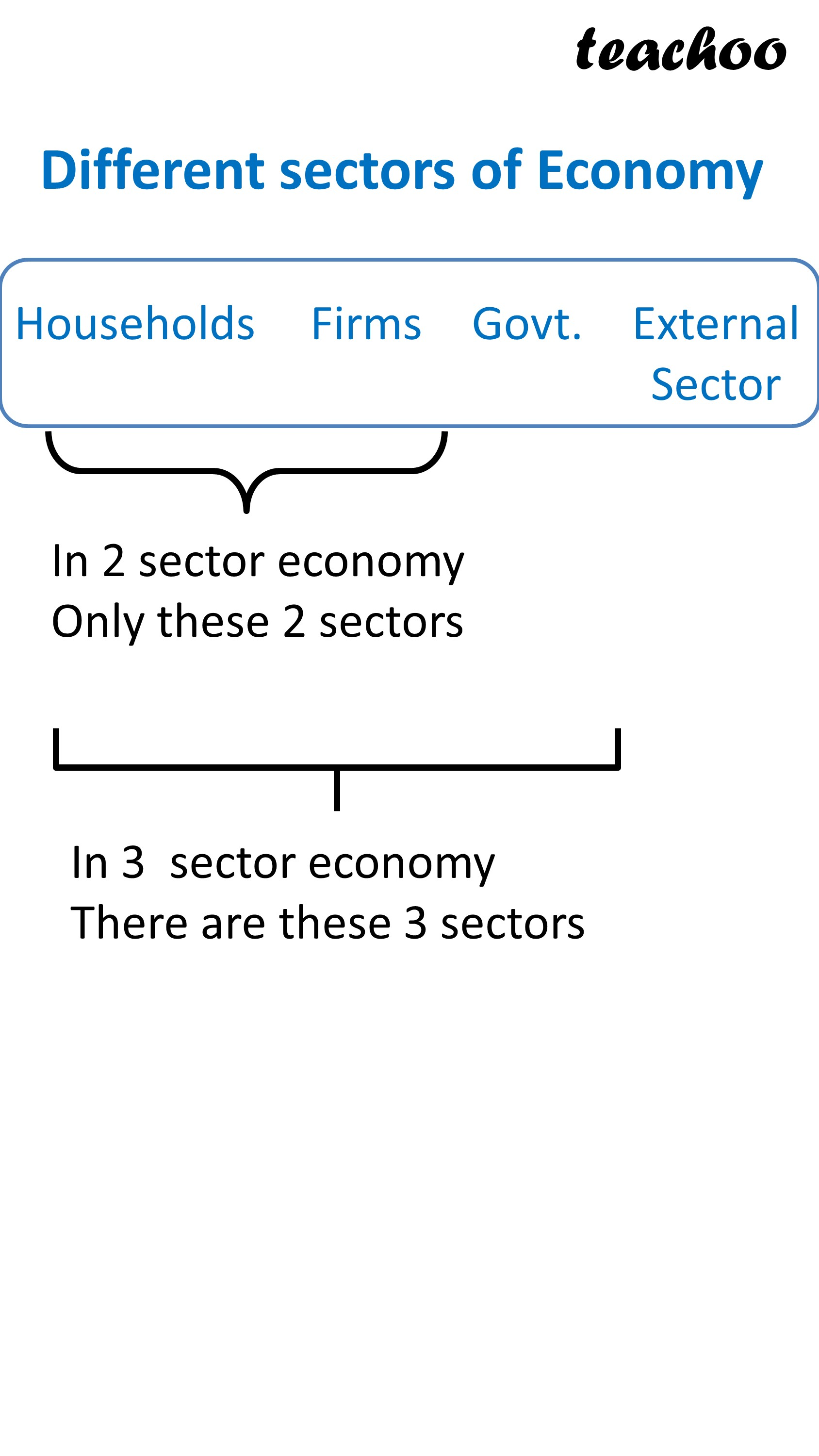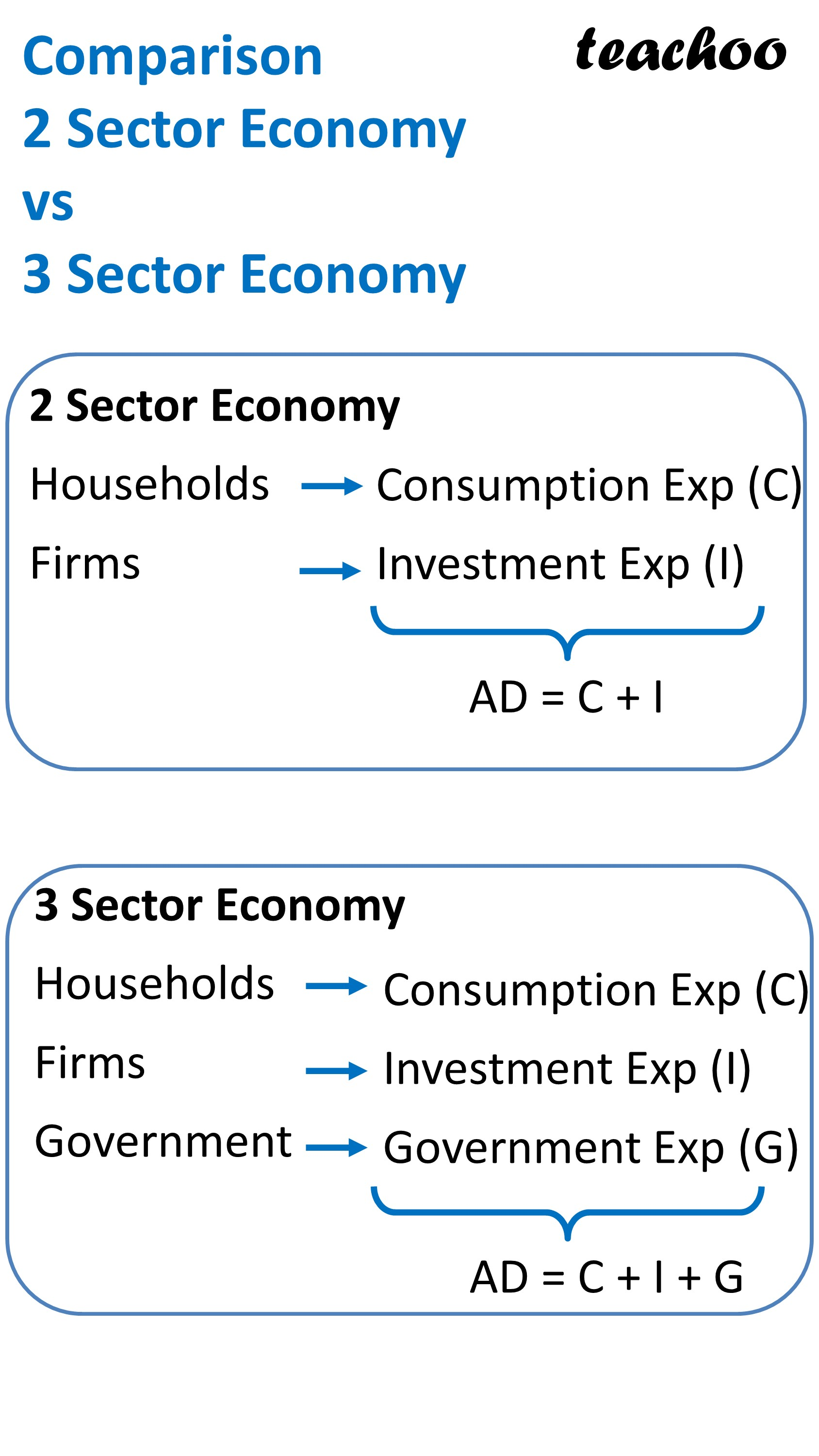## Graph presentation

We know that

On X Axis, we present Income/Output

On Y Axis, we present Aggregate Demand

We can see that

Aggregate Demand is positively sloping starting from Point A

OA Represents Autonomous Expenditure

Now, if Aggregate Demand contains 3 sectors

This is because of Govt Expenditure

It can be seen that

This is because we assume Govt Expenditure also to be constant (it does not change with increase in income)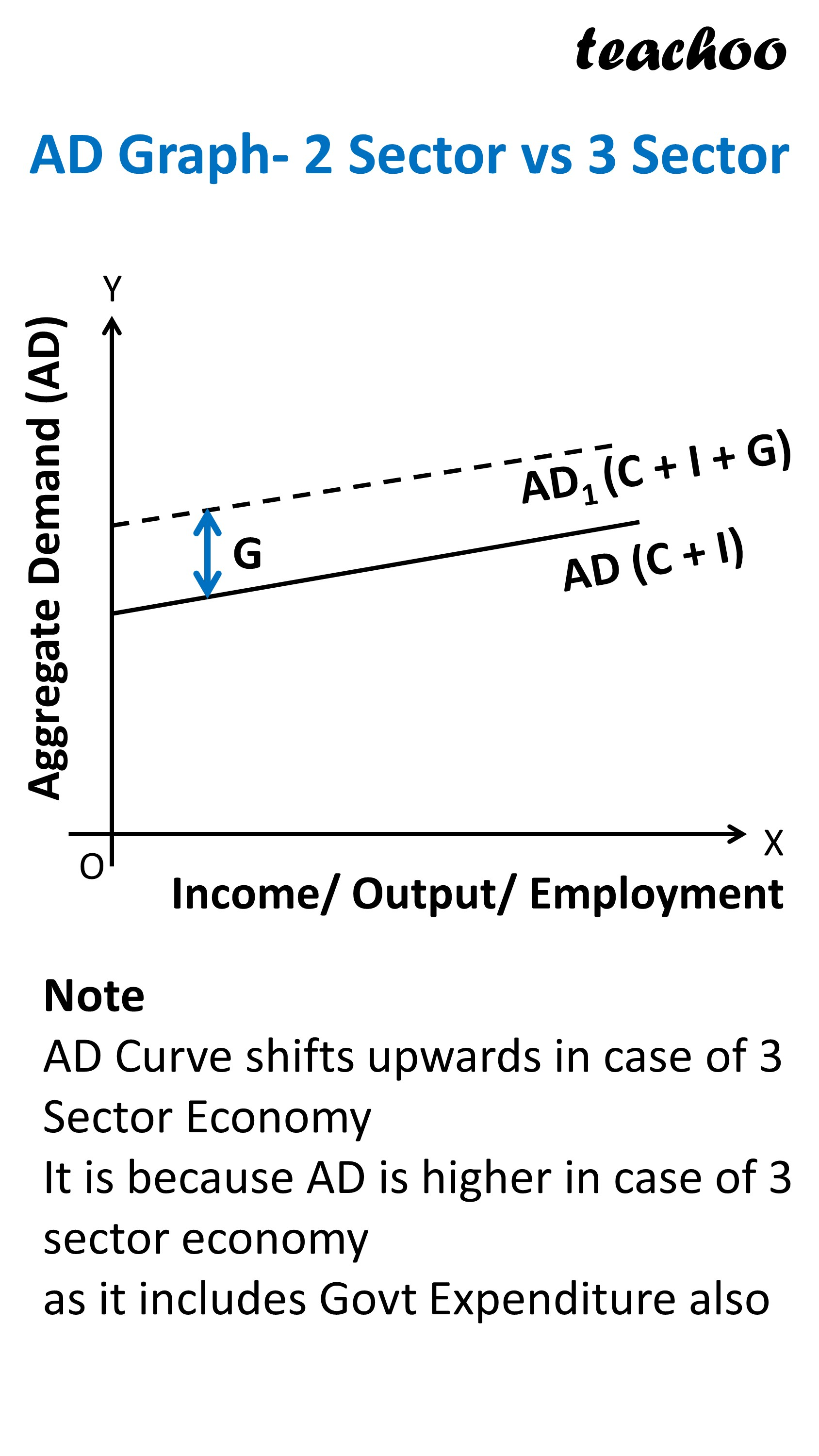## How is Equilibrium Point Determined in Case of 3 Sector Economy?

Just like in normal case

Equilibrium Point is determined at a point where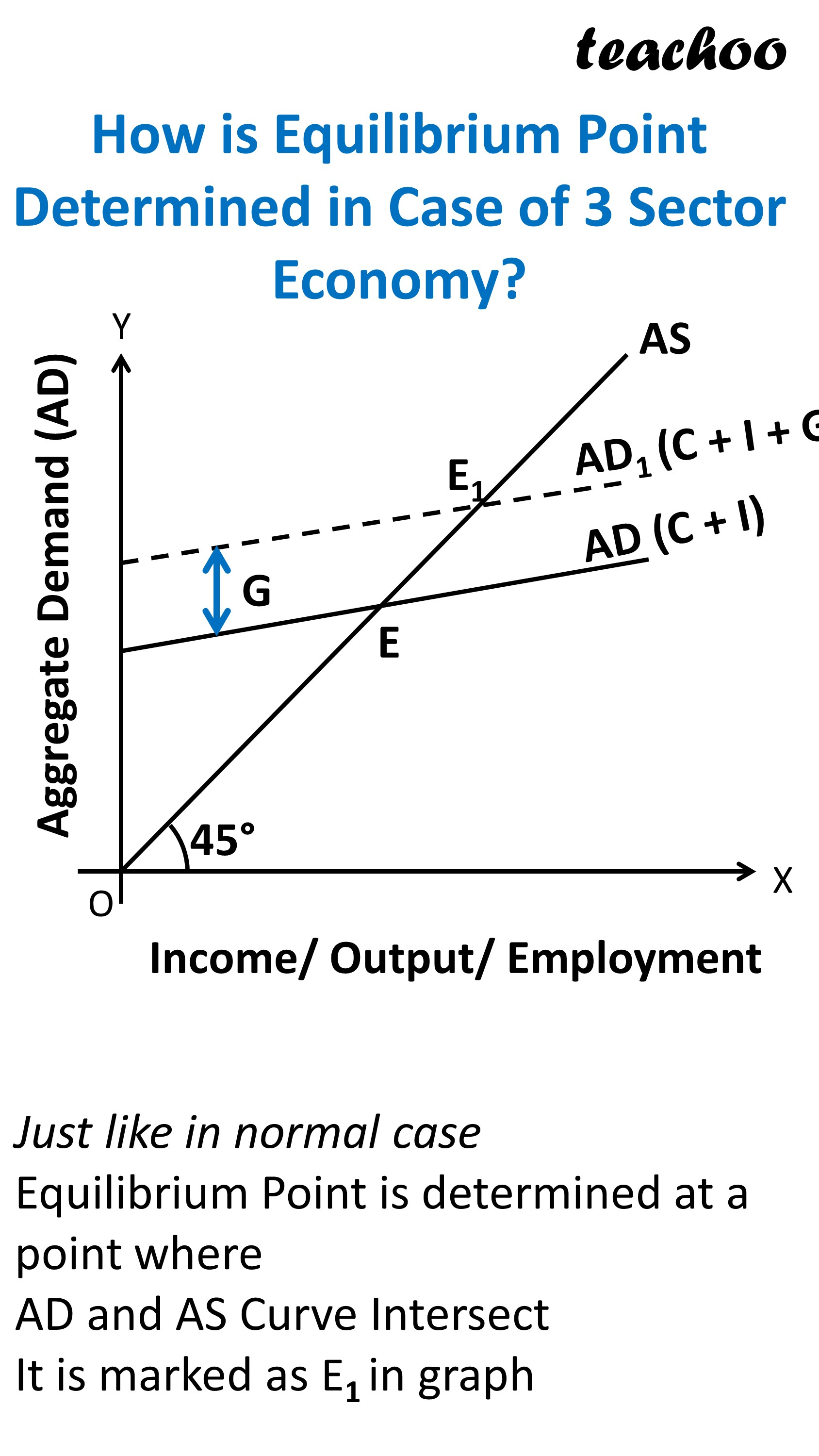On X Axis, we present Income or Output

On Y Axis, we represent Demand

It can be seen that

AD Curve is Positively sloping starting from Point A

AS Curve is also positively sloping starting from Origin (making 45 degree angle with Origin)

Both these curve intersect at Point E which is equilibrium Point

## What happens in case of Excess Demand?

Just like in Normal Case

Excess Demand leads to inflationary gap

Inflationary gap is a situation where

Actual Aggregate Demand exceeds Aggregate Supply

at full employment level

## Graph Presentation

We know that AD and AS Curve normally meet at Point E

This leads to creation of Inflationary Gap (Represented by EF)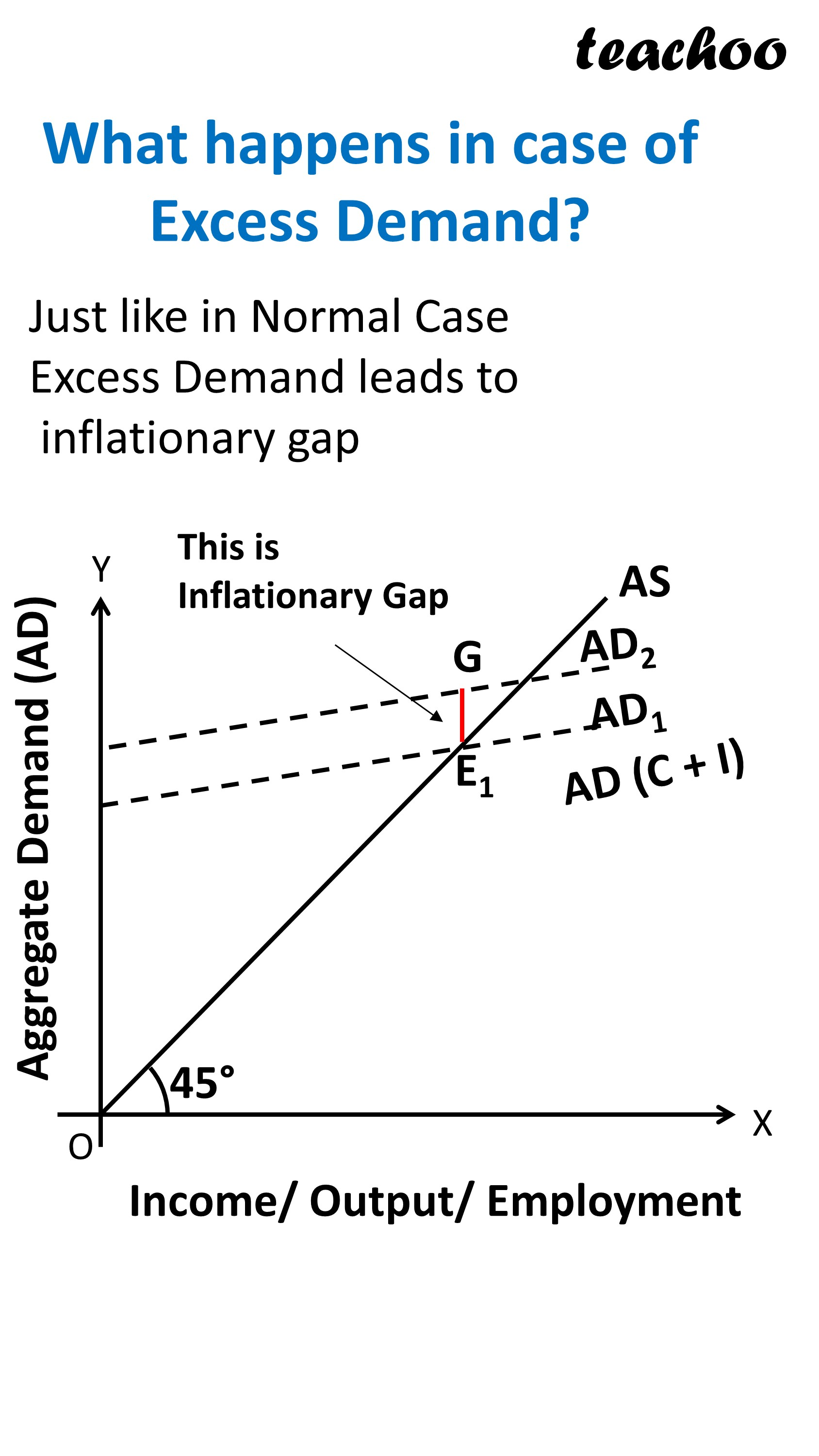## How is it corrected?

It is corrected by Government by reducing Govt Expenditure

It is done up to the amount of Inflationary Gap

This leads to decrease in Aggregate Demand

So AD Curve moves downward till it reaches the full employment equilibrium Point

## What happens in case of Deficient Demand?

Just like in Normal Case

Excess Demand leads to deflationary gap

Deflationary gap is a situation where

Actual Aggregate Demand is less than Aggregate Supply

at full employment level

## Graph Presentation

We know that AD and AS Curve normally meet at Point E

This leads to creation of deflationary Gap (Represented by EF)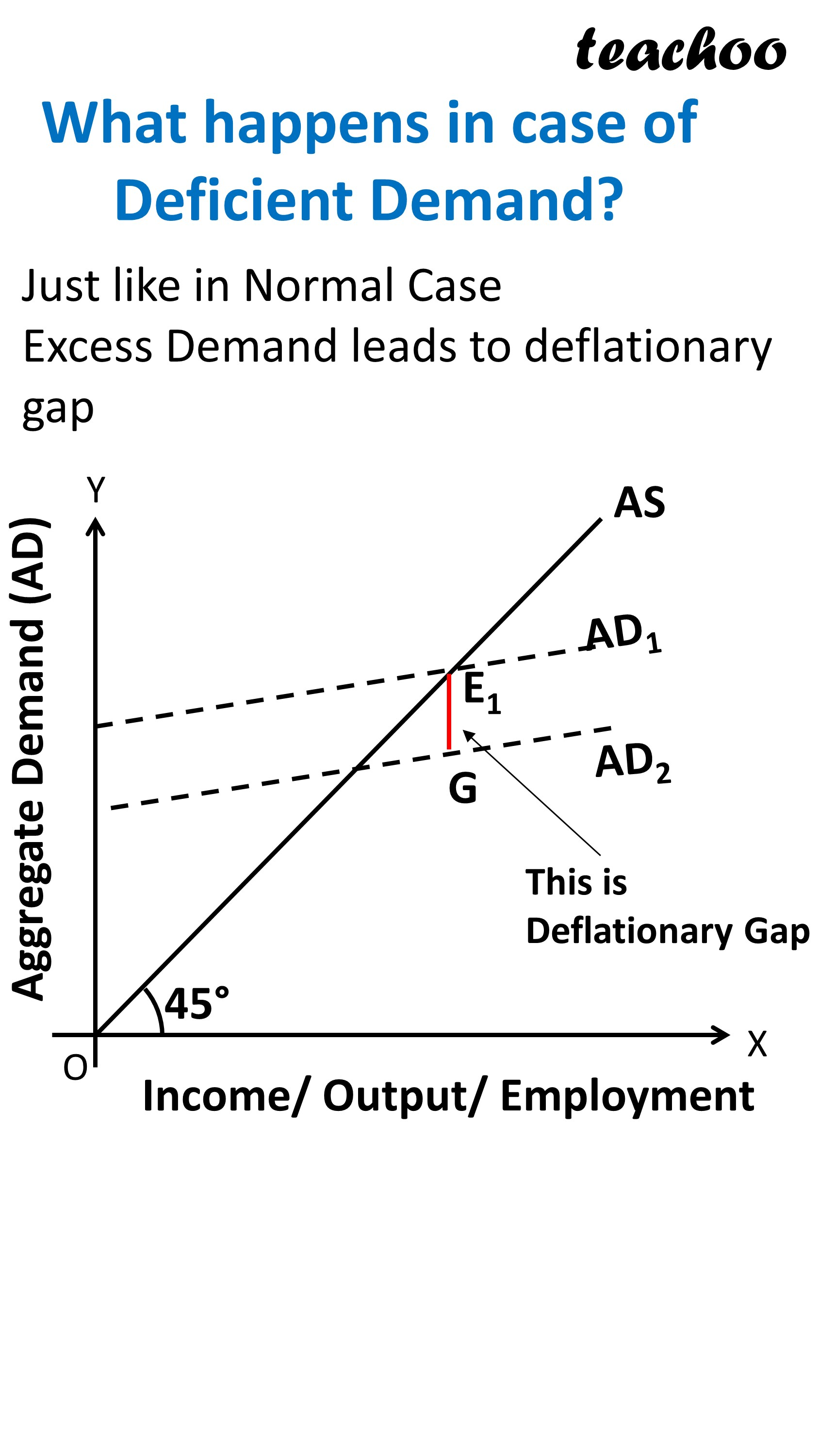## How is it corrected?

It is corrected by Government by Increasing Govt Expenditure

It is done up to the amount of Deflationary Gap

This leads to increase in Aggregate Demand

So AD Curve moves downward till it reaches the full employment equilibrium Point

### NCERT Questions

No questions in this part

### Other Books

#### Question 1

What is 3 sector economy?

#### Question 2

How does government correct excess demand in 3 sector economy?

Get live Maths 1-on-1 Classs - Class 6 to 12

### Transcript

Different sectors of Economy Households Firms External Sector Govt. In 2 sector economy Only these 2 sectors In 3 sector economy There are these 3 sectors Comparison 2 Sector Economy vs 3 Sector Economy 2 Sector Economy Households Firms Consumption Exp (C) Investment Exp (I) AD = C + I 3 Sector Economy Households Firms Government Consumption Exp (C) Investment Exp (I) Government Exp (G) AD = C + I + G AD Graph- 2 Sector vs 3 Sector Aggregate Demand (AD) Income/ Output/ Employment Note AD Curve shifts upwards in case of 3 Sector Economy It is because AD is higher in case of 3 sector economy as it includes Govt Expenditure also How is Equilibrium Point Determined in Case of 3 Sector Economy? Aggregate Demand (AD) Income/ Output/ Employment Just like in normal case Equilibrium Point is determined at a point where AD and AS Curve Intersect It is marked as E1 in graph What happens in case of Excess Demand? Just like in Normal Case Excess Demand leads to inflationary gap This is Inflationary Gap Income/ Output/ Employment What happens in case of Deficient Demand? Just like in Normal Case Excess Demand leads to deflationary gap Income/ Output/ Employment